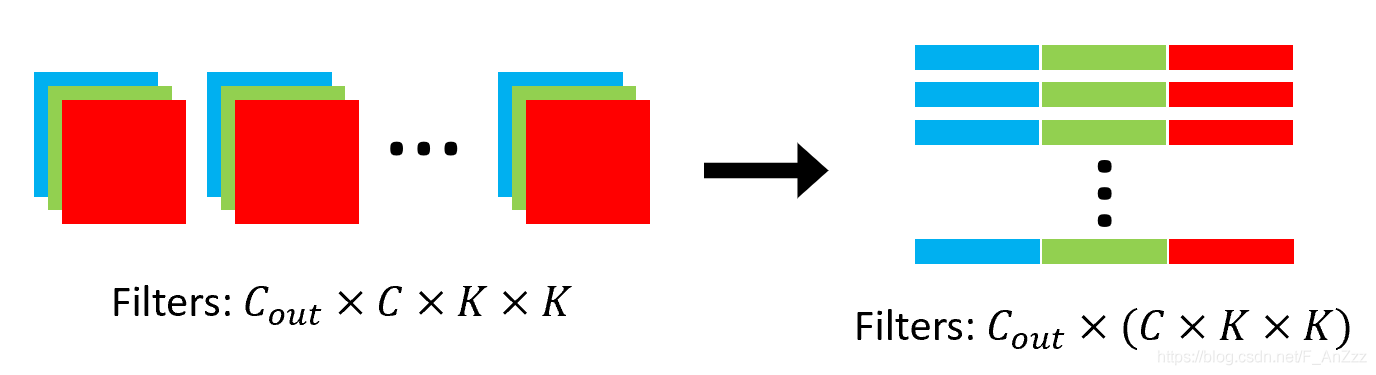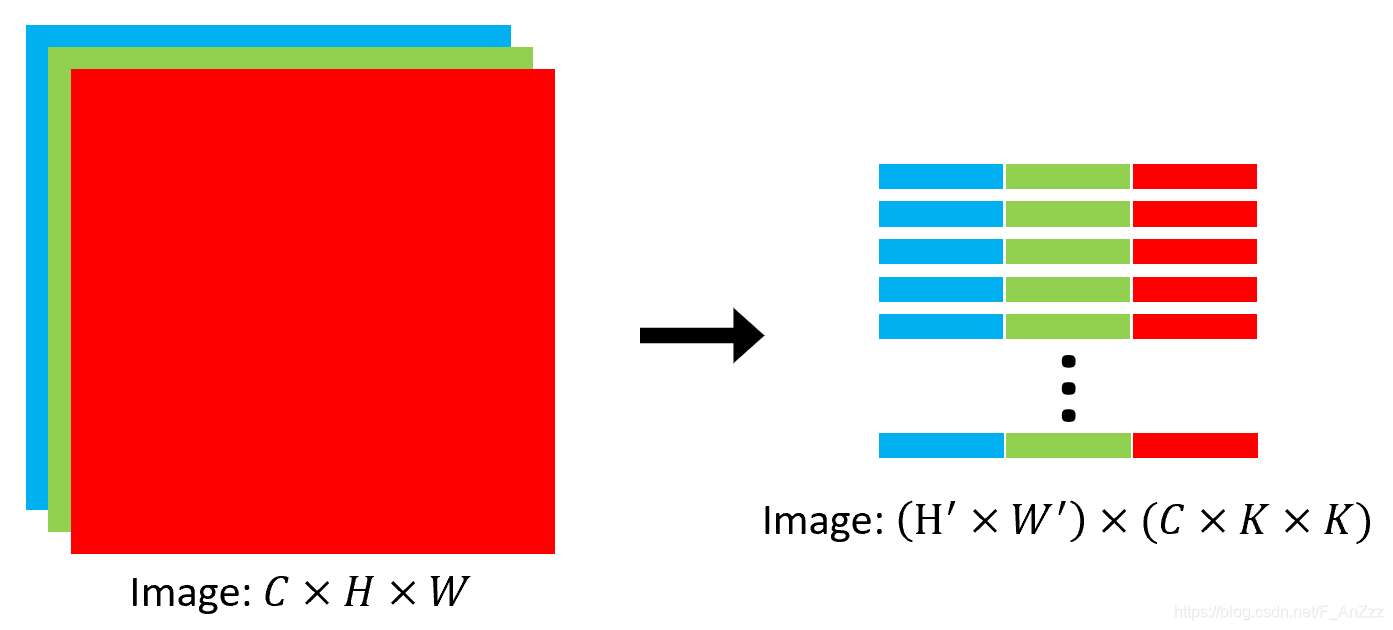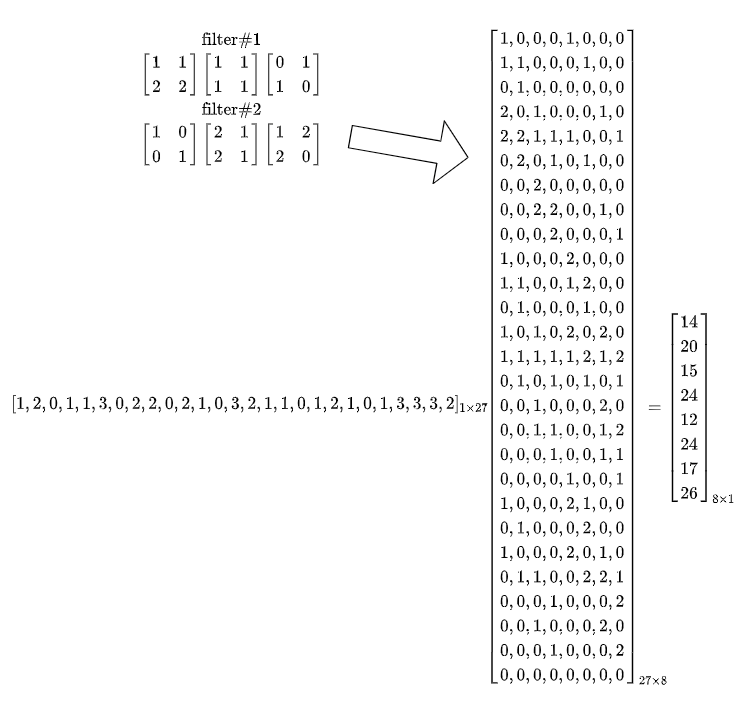## 卷积

### 单通道卷积### 多通道卷积## 多通道卷积计算底层实现

### 第一步：分别将特征图和卷积转换为矩阵

#### 卷积核$$\left[\begin{array}{ll} 1 & 1 \\ 2 & 2 \end{array}\right]\left[\begin{array}{ll} 1 & 1 \\ 1 & 1 \end{array}\right]\left[\begin{array}{ll} 0 & 1 \\ 1 & 0 \end{array}\right]$$

$$\left[\begin{array}{ll} 1 & 0 \\ 0 & 1 \end{array}\right]\left[\begin{array}{ll} 2 & 1 \\ 2 & 1 \end{array}\right]\left[\begin{array}{ll} 1 & 2 \\ 2 & 0 \end{array}\right]$$

#### 特征图$F \times C^{T}=\left(\left(H^{\prime} \times W^{\prime}\right) \times(C \times K \times K)\right) \times\left(C_{\text {out }} \times(C \times K \times K)\right)^{T}=\left(H^{\prime} \times W^{\prime}\right) \times C_{\text {out }}$

  1 2 3 4 5 6 7 8 9 10 11 12 13 14 15 16 17 18  import torch from torch.nn.functional import unfold, fold x = torch.Tensor([[[[1, 2, 0], [1, 1, 3], [0, 2, 2]], [[0, 2, 1], [0, 3, 2], [1, 1, 0]], [[1, 2, 1], [0, 1, 3],[3, 3, 2]]]]) print(x.shape) weight = torch.Tensor([[[[1, 1], [2, 2]], [[1, 1], [1, 1]], [[0, 1], [1, 0]]], [[[1, 0], [0, 1]], [[2, 1], [2, 1]], [[1, 2], [2, 0]]]]) print(weight.shape) kernel_size = 2 F = unfold(x, kernel_size).transpose(1, 2) print(F) C = weight.view(weight.size(0), -1) print(C) # F x C' out_unf = F.matmul(C.t()) print(out_unf) out = fold(out_unf.transpose(1, 2), (2, 2), (1, 1)) print(out) print(out.shape)## 总结与分析

1. 输出层的节点值并不是由全部输入层的节点值与权重乘积和得到，而只有部分输入节点参与到计算；从另一的角度可以当成是有一部分权重为零。
2. 有大量权重被共享，有几个滤波器就共享了几套权重参数，这点原因应该是空间平移不变性，即某一区域和另一区域并没有差别。
3. 上面将所有特征图拉成一维向量，这样只是和全连接层做对比，实际上，因为图像数据存在多通道，例如一般情况下的RGB三通道图像，每个通道包含一个维度的信息；另外图像中像素点之间的位置信息在二维图像中可以更好的呈现（编码），而拉成一维向量会丢失这些信息。
4. 从信息论角度来说，三通道图像是否有图形的信息存在冗余。